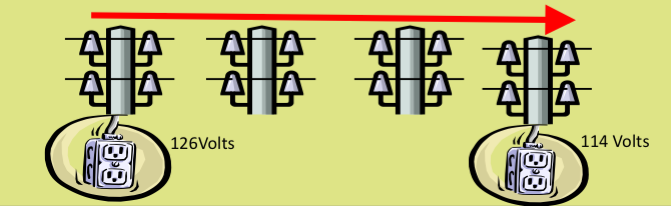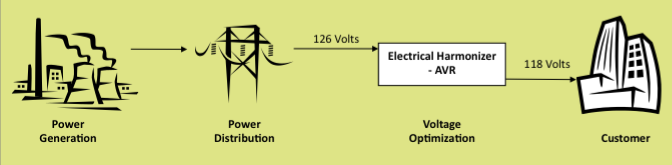# An Energy Problem: Forced Consumption

The problem of force consumption in terms of energy conservation is that of line loss resistance.

Facilities near the beginning of the grid receive voltage at a higher level than needed and waste energy. Over \$1 billion is wasted per year by commercial buildings due to forced grid consumption.Voltage optimization reduces electrical energy consumption by up to 10%, which also reduces the facility’s energy cost and greenhouse gas emissions.Here’s a technical explanation of how voltage optimization is used for power reduction and power savings.

If a 5Ω load resistor is connected to a 100V source it will draw 20A and consume 2kW. If we now put a transformer between the source and the load to reduce the load voltage by 5% to 95V, the load current will be reduced to 19A, with a power consumption of 1805W. This is also the power drawn from the source, which will now be supplying 18.05A. It can be seen that both the load and source currents are lower than they were without the transformer, but at the transformer the voltage has been stepped down (0.95:1) and the current up (1:0.95), so that the power into the transformer is the same as the power out.

As far as the load is concerned a similar reduction could be obtained by adding another resistor in series instead of the transformer.  We would have to pick a value of 0.263Ω to achieve the same result. Now both the load and source currents will be 19A. The load power has still been reduced to 1805W, but the source power has only been reduced to 1900W. This is because, unlike the transformer, the extra resistor is also consuming power (95W).

The Legend Power Harmonizer-AVR system is effectively a low loss transformer with a variable ratio. Both source and load powers are reduced at the same time and the power can be measured either at the source or the load. As in any real connection there will be some losses and in the above example this would be less than 10W. Although the system could be set up to reduce power consumption at any voltage, it is normally used where the line voltage is too high. This means that the consumer is using and paying for more electrical power than needed and the Harmonizer-AVR reduces this consumption to normal levels.

For resistive loads the percentage change in power consumption is approximately twice the percentage change in voltage, for small changes. If we take a per unit approach to the example above a 5% reduction in voltage means the new load voltage is 1 – 0.05. The new power is (1 – 0.05)<sup>2</sup>, which is 1<sup>2</sup> – 2 x 1 x 0.05 + 0.05<sup>2</sup>. This is approximately 1 – 0.10 = 0.9 per unit, a 10% reduction, or 1800W.